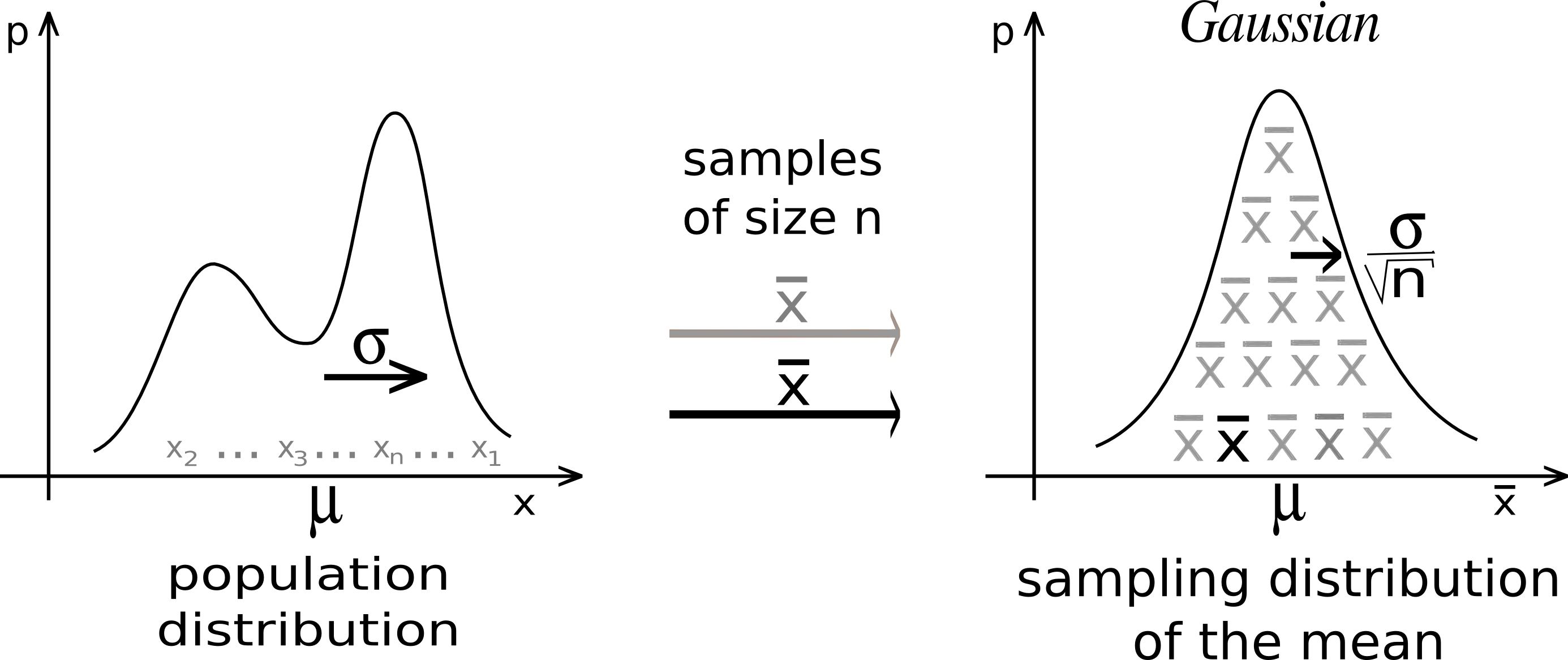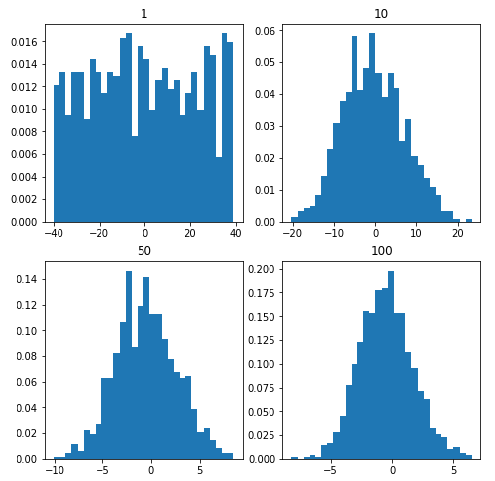# Python – Central Limit Theorem

The definition:

The sample mean will approximately be normally distributed for large sample sizes, regardless of the distribution from which we are sampling.Suppose we are sampling from a population with a finite mean and a finite standard-deviation(sigma). Then
Mean and standard deviation of the sampling distribution of the sample mean can be given as:Whererepresents the sampling distribution of the sample mean of size n each,andare the mean and standard deviation of the population respectively.

The distribution of the sample tends towards the normal distribution as the sample size increases.

Code: Python implementation of the Central Limit Theorem

 import numpy  import matplotlib.pyplot as plt     # number of sample  num = [1, 10, 50, 100]    # list of sample means  means = []       # Generating 1, 10, 30, 100 random numbers from -40 to 40  # taking their mean and appending it to list means.  for j in num:      # Generating seed so that we can get same result       # every time the loop is run...      numpy.random.seed(1)      x = [numpy.mean(          numpy.random.randint(              -40, 40, j)) for _i in range(1000)]      means.append(x)  k = 0    # plotting all the means in one figure  fig, ax = plt.subplots(2, 2, figsize =(8, 8))  for i in range(0, 2):      for j in range(0, 2):          # Histogram for each x stored in means          ax[i, j].hist(means[k], 10, density = True)          ax[i, j].set_title(label = num[k])          k = k + 1

Output:It is evident from the graphs that as we keep on increasing the sample size from 1 to 100 the histogram tends to take the shape of a normal distribution.

Rule of thumb:
Of course, the term “large” is relative. Roughly, the more “abnormal” the basic distribution, the larger n must be for normal approximations to work well. The rule of thumb is that a sample size n of at least 30 will suffice.

Why is this important?
The answer to this question is very simple, as we can often use well developed statistical inference procedures that are based on a normal distribution such as 68-95-99.7 rule and many others, even if we are sampling from a population that is not normal, provided we have a large sample size.

Attention geek! Strengthen your foundations with the Python Programming Foundation Course and learn the basics.

To begin with, your interview preparations Enhance your Data Structures concepts with the Python DS Course.

My Personal Notes arrow_drop_upCheck out this Author's contributed articles.

If you like GeeksforGeeks and would like to contribute, you can also write an article using contribute.geeksforgeeks.org or mail your article to contribute@geeksforgeeks.org. See your article appearing on the GeeksforGeeks main page and help other Geeks.

Please Improve this article if you find anything incorrect by clicking on the "Improve Article" button below.

Article Tags :
Practice Tags :

9

Please write to us at contribute@geeksforgeeks.org to report any issue with the above content.